# Nuclear Physics

Binding Energy : According to Heisenberg atomic model, the nucleus contains protons and neutrons and electrons revolve round the nucleus in different atomic orbits. The experimentally measured mass of nucleus is found to be less than the sum of masses of its constituent particles (protons and neutrons). The difference in mass is known as mass defect.

i.e mass defect (Δ m) = (Zmp + Nmn ) - Mn

where mp = mass of a proton

mn = mass of a neutron

z = number of protons

N = number of neutrons

Mn = experiment mass of nucleus

According to Einstein's mass energy equivalence relation, the disappeared mass (Δ m) has been converted into energy as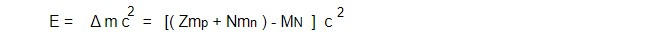This energy equivalent to mass defect which is responsible for keeping the nucleons bound inside the nucleus is known as binding energy.

It is the energy required to dissolve the nucleus into its constituent particles far from each other.

Average Binding energy: It is the energy separated for the individual nucleon given as

Average Binding energy: Total BE/ Total number of nucleon = BE/A

Average Binding energy or Binding energy per nucleons is the energy required to take out either a proton or a neutron from the nucleus.

Binding Energy Curve: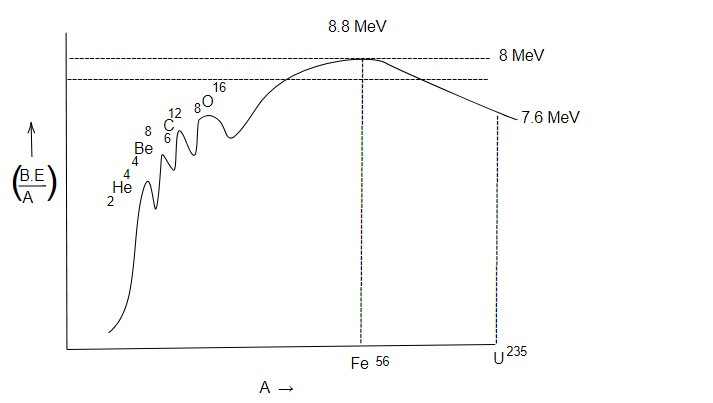The curve obtained by plotting binding energy per nucleon along y axis and atomic mass number along x axis is known as Binding energy curve. This curve gives quantitative description of stability of nucleus. If Binding energy is larger positive, then the nucleus is stable and if binding energy is small or negative, then nucleus is likely to be unstable and radioactive.

Conclusions drawn from Binding energy curve :

1. The Binding energy per nucleus goes on increasing rapidly for lighter elements then becomes maximum and again fall off for heavier elements.

2. The nuclei of intermediate mass have more binding energy per nucleon and relatively more stable. So, when lighter nuclei fuse or heavier nuclei disintegrate to form the nuclei of intermediate mass, then large energy is released.

3. Iron has maximum Binding energy per nucleon which is also reflected by its abundance in nature.

4. Average Binding energy per nucleon is about 8 Mev which shows that Binding energy per nucleon is almost independent to size of nucleus.

5. There are peaks in Binding energy curve for the nuclei whose atomic mass number is integral multiple of 4 (i.e A = 4n)

Nuclei have more Binding energy per nucleon than their neighbours.

6. The nuclei having either proton number (z) or neuron number (N) corresponds to either of magic number 2,8,20,28,50,82,126........ have more Binding energy per nucleon and more stable.

Nuclear Stability

Out of 900 different isotopes found in nature, only about 272 of them are found to be stable. The nuclei having both even number of neutrons and protons are found. Most stable or flavoured by the nature and they are most stable. The nuclei with even odd combination of neutrons and proton found stable are around fifty. And they are intermediate in stability. The odd odd combination of neutrons and proton makes the nuclei least stable since stable nuclei in this combination are least.

The number of neutrons and protons found in stable nuclei are plotted in graph which is known as stability curve or Segre chart.

For lighter element weight atomic number z<20 only these nuclei having N/Z =1 i.e proton and neutron number equal are likely to be stable.Those having ratio N/Z line above or below this line are unstable. For heavier nuclei the repulsive force between large number of proton decreases the nuclear attractive force and so to stable heavier nuclei must have greater number of neutrons than protons.

For stable nuclei, the number of neutrons must be upto a optimim value.

When the optimum number of neutrons required for stability is plotted against number of protons, then a region also known as stability region is found only those nuclei lying within this region are stable and lying above or below the region are unstable. Nuclei whose ratio N/Z lies in fringe of region are artificial radioactive nuclei.

Nuclear force

Nuclear contains only protons and neutrons. The Electrostatics repulsive force between positively charged protons is much greater than the attractive gravitational force. So, the repulsive force should have disrupt the nucleus for this. A third type of attractive force has to arise which is nuclear force. Nuclear force exists between only two nucleons i.e force exists between proton - proton, neutron-neutron and proton-neutron.

Properties of Nuclear force:

1. Nuclear force is short range force

Nuclear force between any two nucleons exists if the separation of them is about 2-2 fermi. Beyond this range (about 4 fermi), the nuclear force vanishes. When the nucleons approach nearer than about 1.4 fermi, they start repelling each other which prevents the nucleus from being collapsed.

2. Nuclear force is charge independent

There are two hypothesis existing about the strength of nuclear force between nucleons.

a. Hypothesis of charge independent

According to this hypothesis, the nuclear force between any two nucleons is same irrespective of their charges, i.e nuclear force between n-n ≈ p-p ≈n-p.

This hypothesis is verified on the basis of comparative study of Binding energy of hydrogen and helium. They are mirror nuclei having same atomic mass number but number of protons and neutrons interchanged. The Binding energy of Hydrogen is 8.5 MeV whereas that of helium is 7.7 MeV.

In tritium, there are two (n-p) interaction and more (n-n) interaction but in helium, there are two (n-p) and one (p-p) interaction. If 0.8 MeV energy between protons due to Binding energy comes out to be same. This clearly indicates that the force between nucleons is independent to their charges.

b. Hypothesis of charge symmetry

This hypothesis states that the nuclear force between two similar particles is same but it is less than the dissimilar particle i.e interaction force between n-n ≈ p-p ≈n-p.

This hypothesis is verified on the basis of comparative study of Binding energy of isobars Boron, Carbon, Beryllium has 4(n-p) pairs and 1(n-n) pair whereas carbon has 4(n-p) pair and 1(p-p) pair. But the Binding energy of both isobars is same which indicates that the nuclear force between (n-n) ≈force between (p-p). Boron has 5(n-p) pairs which has 1(n-p) pair different from others. But the Binding energy of Boron is slightly greater than that of Be and C. Thus indicates that strength of force between (n-p) is slightly greater than between (n-n) and (p-p).

3. Nuclear force is exchangeable force (Meson theory of Nuclear force

According to Yukawa's Meson theory of Nuclear force, the nuclear force between protons rises due to the exchange of π mesons. π Mesons are the particles having mass about 270 times mass of electrons spin zero and having charge equal to that of electrons or zero i.e π+ and π- meson have charge equal to that of electrons in magnitude but π is neutral.

The proton and neutrons are surrounded by the clouds of virtual π mesons. The proton and neutrons are surrounded by π mesons of opposite charges otherwise they are the same particles in different charge states.

4. Nuclear force has saturation property

The nuclear force is limited within a short range of distance between two nucleons. Each nucleon inside nucleus interacts only with its nearest neighbour nucleons but not with all nucleons present. This property of nuclear force is known as Saturation property.

In any nucleus having atomic mass number A if each nucleon is assumed to interact with remaining (A-1) nucleons, then there are A(A-1)/2 total interaction pairs. Since interaction gives rise to nuclear force, the binding energy must be proportions to number of interaction pairs.

i.e BE A(A-1)/2

=> BE A^2

But Binding energy is found to vary directly on A. This concludes that the nuclear force exists only between nearest neighbour nucleons.

5. Nuclear force is non central force

The nuclear force doesn't obey inverse square law and doesn't direct along the line joining two nucleons. So it is non central force which rather depends on relative orientation of nucleons with each other.

6. Nuclear force is spin dependent

The nuclear force between nucleons having parallel spin is greater than between nucleons having anti parallel spin so it depends on total spin of the particles.

Nuclear Model

Liquid drop model:

This model of nucleus was proposed by Neil Bohr according to which the nucleus is assumed as a electrically charged liquid drop having constant density but of varying mass similarities between liquid drop and nucleus.

Assumption of Liquid drop model

1. The shape of liquid drop is spherical a equilibrium due to symmetric forces of surface tension. Similarly, the shape of nucleus is also spherical in stable state due to nuclear force.

2. The mass and volume of nucleus is directly proportional to the atomic mass number (A) but density is independent to A. Likewise the mass of liquid drop depends on volume or number of molecules but density is independent to overall size of liquid state.

3. The nucleons in nucleus play the same role as molecule does in liquid drop. The intermolecular force of attraction between molecules and nuclear force between nucleons ate same, both being short ranged force and having tendency to saturate.

4. The nucleons moves within the spherical enclosure due to nuclear potential barrier similar to the movement of molecules within liquid drop under the effect of surface tension.

5. The binding energy per nucleon for the nuclei is constant (about 8 MeV) which is analogous to the latent heat of vapourisation of liquid constant for different drops of liquid.

6. The liquid drop evaporates on raising the temperature due to increase in thermal energy. Similarly when energy is given to the nucleus (by bombarding it energetic projectile particles), then a compound nucleus is formed in excited state which emits nuclear radiation return into stable ground state.

7. When the liquid drop gains external energy, then the spherical shape of nucleus changed into ellipsoidal and finally breaks up into smaller drops. In a similar manner, the nucleus drop changes its shape from spherical to ellipsoidal and then dumb bell and finally breaks up into smaller nuclei. If it gains extra energy. The process is nuclear fission.

Bohr and wheeler theory of nuclear fission on the basis of liquid drop model.

Bohr and wheeler successfully explained the nuclear fission process on the basis of liquid drop model.

The nuclear fission of Uranium when bombarded by a neutron is as given by the reaction.The liquid drop is spherical in shape at equilibrium due to intermolecular force of attraction. When the energy is given to the liquid drop, then it oscillates in number of ways. The oscillation of molecules tends to distort the spherical shape whereas surface tension tends to maintain the spherical shape. If the external energy is sufficient then the spherical shape. if the external energy is sufficient then the spherical shape changes into ellipsoidal and then dumb bell shape and finally single larger drops breaks up into smaller liquid drops. Similarly, the shape of nucleus is also spherical at stable equilibrium. The attractive nuclear force tends to maintain the spherical shape whereas the repulsive force between protons tends to change the shape. When energy is applied from outside to the nucleus, then the compound nucleus is formed at excited state. Then the spherical shape of nucleus changes into the ellipsoidal and then into dumb bell shaped. The two bells of dumb bell are positively charge and when the repulsive force exceeds the nuclear force. the bells get separated. So, smaller nuclei are formed which is known as nuclear fission.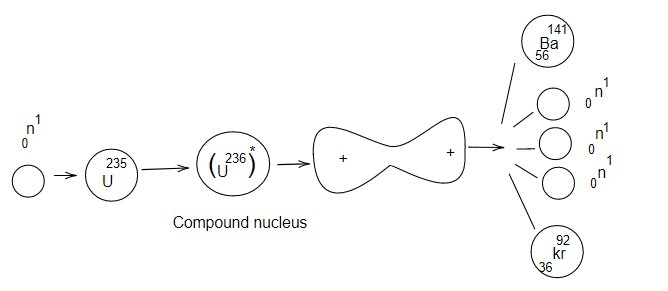Weizsacher's Semi empirical mass formula

On the basis of liquid drop model, Weizsacher's gave the relation to find the mass of nucleus which is known as Weizsacher's semi empirical mass formula. He found the binding energy of nucleus very accurately by defining different energy term that contribute to total binding energy. This formula is in explaining the stability of nucleus and β decay process by unstable nucleus.

The different energy terms

1. Volume energy term (Bv):

When the liquid evaporates completely, then the heat energy supplied is used up in breaking the molecular bonds. So, heat energy required is equivalent to binding energy of liquid drop.

i.e BE = LM = L(nm) where L = latent heat of vapourisation

n= total number of molecules in drop

m= mass of a molecule

M=total mass of liquid drop

BE ∝ n

By analogy with the liquid drop, the binding energy in the nucleus is directly proportional to the total number of nucleons (A). Since atomic mass number (A) is directly proportional to the volume of nucleus, BE also varies directly with volume. So, this term is also known as volume energy term (Bv)

ie. Bv ∝ A

or, Bv = avA.......1

where av is coefficient of volume energy term and has value 14 MeV.

2. Surface energy term (Bs):

The volume energy term is defined on the assumption that all nucleons are equally surrounded by other nucleons and the nuclear force saturates. But for the surface nucleons that lie outside the interior nucleons ans so nuclear force doesn't saturate. This is why the volume energy term has to be corrected by an amount that is proportional to surface nucleons. So this term i.e surface energy term (Bs) ∝ number of surface nucleons

or, Bs ∝ surface area = 4 πR^2where as is coefficient of surface energy term and value 13 MeV.

3. Coulomb energy term:

The nucleus contains z protons and +ze protonic charge. So there is Coulomb repulsive force between proton. The energy equivalent to work done against repulsion has to be spent for keeping the protons together. The energy is known as Coulomb energy term which has negative contribution to total BE coulomb energy term and is given as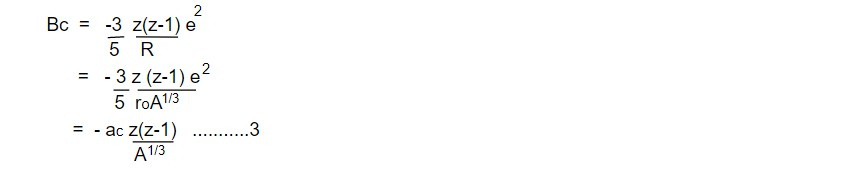where ac is constant of Coulomb energy and has value 0-6 MeV

4. Asymmetry energy term: In lighter nuclei, the number of neutron (N) is equal to number of protons. But in heavier nuclei N>Z due to asymmetriy between number of protons and number of neutrons, the total binding energy is decreased. This energy due to asymmetry of protons and neutrons is known as asymmetry energy term.

This energy is directly proportional to square of excess of neutrons over protons and inversely proportional to atomic mass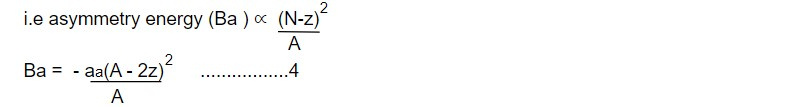where aa is constant of asymmetry energy term and has value 19MeV.

5. Pairing energy term: The nuclei having even even combination protons and neutrons are most abundant and stable in nature. Those having odd even or even odd combination are intermediate in stability. The nuclei having odd protons and odd neutrons are least stable. This indicates that the nucleons tend to exist in pair. The binding energy due to pairing of nucleons is given as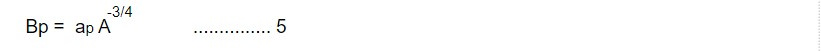where ap is constant of pairing energy term and has value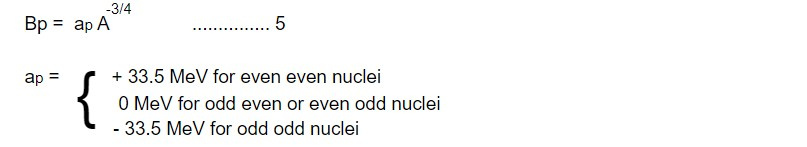Total Binding energy of a nucleus is given by the sum of all energy terms.This formula is also known as Weizsacher's binding energy formula.

The mass of nucleus is given asThis formula is known as Weizsacher's semi empirical mass formula.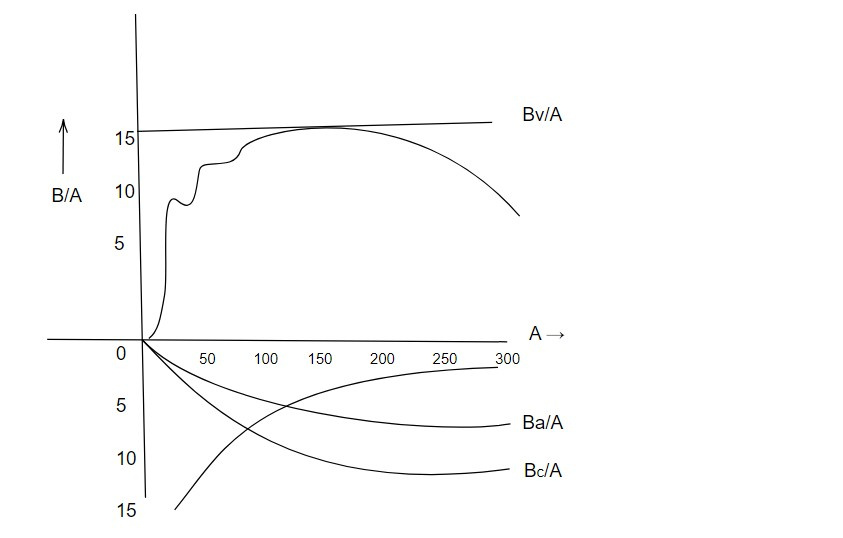Achievements of Liquid drop model

The Liquid Drop Model is a model used to explain the structure and stability of atomic nuclei. It was developed by Niels Bohr and John A. Wheeler in 1939 and is based on the idea that the nucleus can be thought of as a drop of incompressible liquid.

1. This model can estimates the binding energy and nuclear mass more accurately.

2. This model successfully explains the β decay among isobaric family.

3. This model explains the stability of nucleus.

4. This model also explains the nuclear fission and radioactivity.

Failure of Liquid drop model

1. It couldn't explain the stability of nucleus with magic number.

2. It couldn't explain the major magnetic moment and electric quadrupole moment and nuclear spin of a nucleus.

3. It couldn't explain the emission of β particle from a nucleus even they explain β decay.

4. It couldn't explain the spectrum of different particles like α, β and γ in term of their ranges.

This note is taken from Nuclear Physics, BSC physics, Nepal.

This note is a part of the Physics Repository.